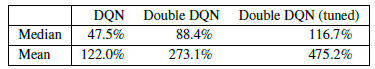# 单智能体强化学习算法

## Double DQN：Deep Reinforcement Learning with Double Q-learning

Posted by MY on August 4, 2020

## 一、问题

$y_{j}=\left\{\begin{array}{ll} R_{j} & \text {is_end}_{j} \text { is true} \\ R_{j}+\gamma \max _{a^{\prime}} Q^{\prime}\left(\phi\left(S_{j}^{\prime}\right), A_{j}^{\prime}, w^{\prime}\right) & \text {is_end}_{j} \text { is false } \end{array}\right.$

## 二、解法

DDQN和Nature DQN一样，也有一样的两个Q网络结构。在Nature DQN的基础上，通过解耦目标Q值动作的选择和目标Q值的计算这两步，来消除过度估计的问题。

Nature DQN对于非终止状态，其目标Q值的计算式子是：

$y_{j}=R_{j}+\gamma \max _{a^{\prime}} Q^{\prime}\left(\phi\left(S_{j}^{\prime}\right), A_{j}^{\prime}, w^{\prime}\right)$

$a^{\max }\left(S_{j}^{\prime}, w\right)=\arg \max _{a^{\prime}} Q\left(\phi\left(S_{j}^{\prime}\right), a, w\right)$

$y_{j}=R_{j}+\gamma Q^{\prime}\left(\phi\left(S_{j}^{\prime}\right), a^{\max }\left(S_{j}^{\prime}, w\right), w^{\prime}\right)$

$y_{j}=R_{j}+\gamma Q^{\prime}\left(\phi\left(S_{j}^{\prime}\right), \arg \max _{a^{\prime}} Q\left(\phi\left(S_{j}^{\prime}\right), a, w\right), w^{\prime}\right)$

## 三、实验内容## 五、优点

DDQN算法出来以后，在处理高偏差方面取得了比较好的效果，因此得到了比较广泛的应用。

##### Share